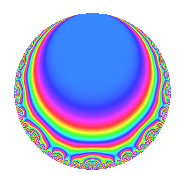# Properties

 Label 164.2.a.aLevel 164 Weight 2 Character orbit 164.a Self dual Yes Analytic conductor 1.310 Analytic rank 0 Dimension 4 CM No Inner twists 1

# Related objects

## Newspace parameters

 Level: $$N$$ = $$164 = 2^{2} \cdot 41$$ Weight: $$k$$ = $$2$$ Character orbit: $$[\chi]$$ = 164.a (trivial)

## Newform invariants

 Self dual: Yes Analytic conductor: $$1.30954659315$$ Analytic rank: $$0$$ Dimension: $$4$$ Coefficient field: 4.4.25808.1 Coefficient ring: $$\Z[a_1, \ldots, a_{5}]$$ Coefficient ring index: $$1$$ Fricke sign: $$-1$$ Sato-Tate group: $\mathrm{SU}(2)$

## $q$-expansion

Coefficients of the $$q$$-expansion are expressed in terms of a basis $$1,\beta_1,\beta_2,\beta_3$$ for the coefficient ring described below. We also show the integral $$q$$-expansion of the trace form.

 $$f(q)$$ $$=$$ $$q + ( -\beta_{1} + \beta_{2} ) q^{3} + ( 2 - \beta_{2} + \beta_{3} ) q^{5} + ( -1 + \beta_{1} + \beta_{2} - \beta_{3} ) q^{7} + ( 3 - \beta_{2} - \beta_{3} ) q^{9} +O(q^{10})$$ $$q + ( -\beta_{1} + \beta_{2} ) q^{3} + ( 2 - \beta_{2} + \beta_{3} ) q^{5} + ( -1 + \beta_{1} + \beta_{2} - \beta_{3} ) q^{7} + ( 3 - \beta_{2} - \beta_{3} ) q^{9} + ( 1 + \beta_{1} - \beta_{2} - \beta_{3} ) q^{11} + 2 \beta_{1} q^{13} + ( -1 - 2 \beta_{1} + 3 \beta_{3} ) q^{15} + 2 \beta_{3} q^{17} + ( 2 - \beta_{1} - \beta_{2} ) q^{19} + ( -2 + 2 \beta_{1} + \beta_{2} - 3 \beta_{3} ) q^{21} + ( -2 - 2 \beta_{2} ) q^{23} + ( 3 - 2 \beta_{1} ) q^{25} + ( -5 - 2 \beta_{1} + 4 \beta_{2} - \beta_{3} ) q^{27} + ( -2 - 2 \beta_{1} + 2 \beta_{2} ) q^{29} + ( -2 \beta_{2} + 2 \beta_{3} ) q^{31} + ( -8 - 2 \beta_{1} + 5 \beta_{2} - \beta_{3} ) q^{33} + ( -7 + 4 \beta_{1} - \beta_{3} ) q^{35} + ( 4 - \beta_{2} - \beta_{3} ) q^{37} + ( -6 + 2 \beta_{1} ) q^{39} - q^{41} + ( 2 \beta_{1} - 2 \beta_{3} ) q^{43} + ( 6 + 2 \beta_{1} - 7 \beta_{2} + 3 \beta_{3} ) q^{45} + ( -2 - 3 \beta_{1} + \beta_{2} ) q^{47} + ( 5 - 4 \beta_{1} + \beta_{2} + 3 \beta_{3} ) q^{49} + ( 4 + 2 \beta_{1} - 6 \beta_{2} + 4 \beta_{3} ) q^{51} + ( -4 + 2 \beta_{1} - 2 \beta_{2} - 2 \beta_{3} ) q^{53} + ( 1 + 4 \beta_{1} - 4 \beta_{2} - \beta_{3} ) q^{55} + ( -4 \beta_{1} + 3 \beta_{2} + \beta_{3} ) q^{57} + ( 2 + 2 \beta_{2} ) q^{59} + ( 6 + 2 \beta_{1} ) q^{61} + ( -6 - \beta_{1} + 3 \beta_{2} - 4 \beta_{3} ) q^{63} + ( -2 + 4 \beta_{1} + 2 \beta_{2} - 4 \beta_{3} ) q^{65} + ( 7 - \beta_{1} + \beta_{2} + \beta_{3} ) q^{67} + ( -6 + 2 \beta_{3} ) q^{69} + ( -4 + \beta_{1} + 3 \beta_{2} - 4 \beta_{3} ) q^{71} + ( 4 - \beta_{2} + 3 \beta_{3} ) q^{73} + ( 6 - 5 \beta_{1} + 3 \beta_{2} ) q^{75} + ( 2 - 4 \beta_{1} + \beta_{2} + \beta_{3} ) q^{77} + ( -6 - \beta_{1} + 3 \beta_{2} ) q^{79} + ( 7 + 6 \beta_{1} - 3 \beta_{2} - 3 \beta_{3} ) q^{81} + ( -2 - 4 \beta_{1} + 2 \beta_{3} ) q^{83} + ( 4 - 4 \beta_{1} + 6 \beta_{2} - 2 \beta_{3} ) q^{85} + ( 12 + 2 \beta_{1} - 4 \beta_{2} - 2 \beta_{3} ) q^{87} + ( -4 \beta_{1} + 2 \beta_{2} ) q^{89} + ( 12 - 2 \beta_{1} + 6 \beta_{3} ) q^{91} + ( -2 - 4 \beta_{2} + 6 \beta_{3} ) q^{93} + ( 7 - 2 \beta_{1} - 4 \beta_{2} + 3 \beta_{3} ) q^{95} + ( 2 - 4 \beta_{3} ) q^{97} + ( 16 + 7 \beta_{1} - 7 \beta_{2} - 4 \beta_{3} ) q^{99} +O(q^{100})$$ $$\operatorname{Tr}(f)(q)$$ $$=$$ $$4q + 2q^{3} + 4q^{5} + 12q^{9} + O(q^{10})$$ $$4q + 2q^{3} + 4q^{5} + 12q^{9} + 4q^{11} - 10q^{15} - 4q^{17} + 6q^{19} - 12q^{23} + 12q^{25} - 10q^{27} - 4q^{29} - 8q^{31} - 20q^{33} - 26q^{35} + 16q^{37} - 24q^{39} - 4q^{41} + 4q^{43} + 4q^{45} - 6q^{47} + 16q^{49} - 4q^{51} - 16q^{53} - 2q^{55} + 4q^{57} + 12q^{59} + 24q^{61} - 10q^{63} + 4q^{65} + 28q^{67} - 28q^{69} - 2q^{71} + 8q^{73} + 30q^{75} + 8q^{77} - 18q^{79} + 28q^{81} - 12q^{83} + 32q^{85} + 44q^{87} + 4q^{89} + 36q^{91} - 28q^{93} + 14q^{95} + 16q^{97} + 58q^{99} + O(q^{100})$$

Basis of coefficient ring in terms of a root $$\nu$$ of $$x^{4} - 10 x^{2} - 6 x + 9$$:

 $$\beta_{0}$$ $$=$$ $$1$$ $$\beta_{1}$$ $$=$$ $$\nu$$ $$\beta_{2}$$ $$=$$ $$($$$$\nu^{3} - 7 \nu - 3$$$$)/3$$ $$\beta_{3}$$ $$=$$ $$($$$$-\nu^{3} + 3 \nu^{2} + 7 \nu - 12$$$$)/3$$
 $$1$$ $$=$$ $$\beta_0$$ $$\nu$$ $$=$$ $$\beta_{1}$$ $$\nu^{2}$$ $$=$$ $$\beta_{3} + \beta_{2} + 5$$ $$\nu^{3}$$ $$=$$ $$3 \beta_{2} + 7 \beta_{1} + 3$$

## Embeddings

For each embedding $$\iota_m$$ of the coefficient field, the values $$\iota_m(a_n)$$ are shown below.

For more information on an embedded modular form you can click on its label.

Label $$\iota_m(\nu)$$ $$a_{2}$$ $$a_{3}$$ $$a_{4}$$ $$a_{5}$$ $$a_{6}$$ $$a_{7}$$ $$a_{8}$$ $$a_{9}$$ $$a_{10}$$
1.1
 0.707500 3.31526 −2.46810 −1.55466
0 −3.24028 0 2.56613 0 −0.858626 0 7.49944 0
1.2 0 0.0950939 0 1.17025 0 3.14501 0 −2.99096 0
1.3 0 2.21551 0 3.59669 0 −5.06479 0 1.90849 0
1.4 0 2.92968 0 −3.33307 0 2.77840 0 5.58303 0
 $$n$$: e.g. 2-40 or 990-1000 Significant digits: Format: Complex embeddings Normalized embeddings Satake parameters Satake angles

## Inner twists

This newform does not admit any (nontrivial) inner twists.

## Atkin-Lehner signs

$$p$$ Sign
$$2$$ $$-1$$
$$41$$ $$1$$

## Hecke kernels

There are no other newforms in $$S_{2}^{\mathrm{new}}(\Gamma_0(164))$$.